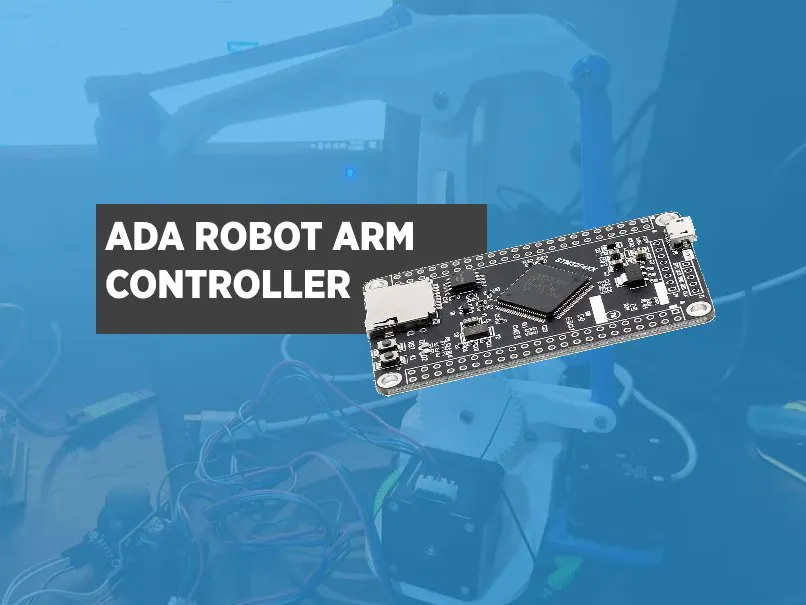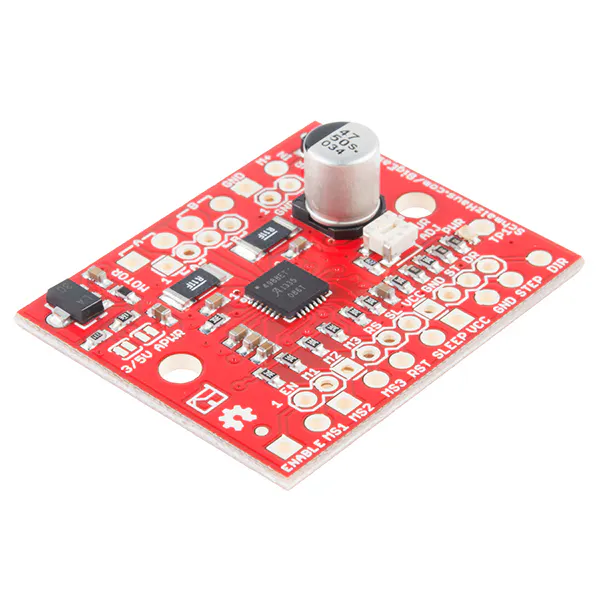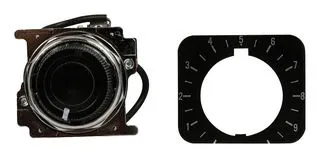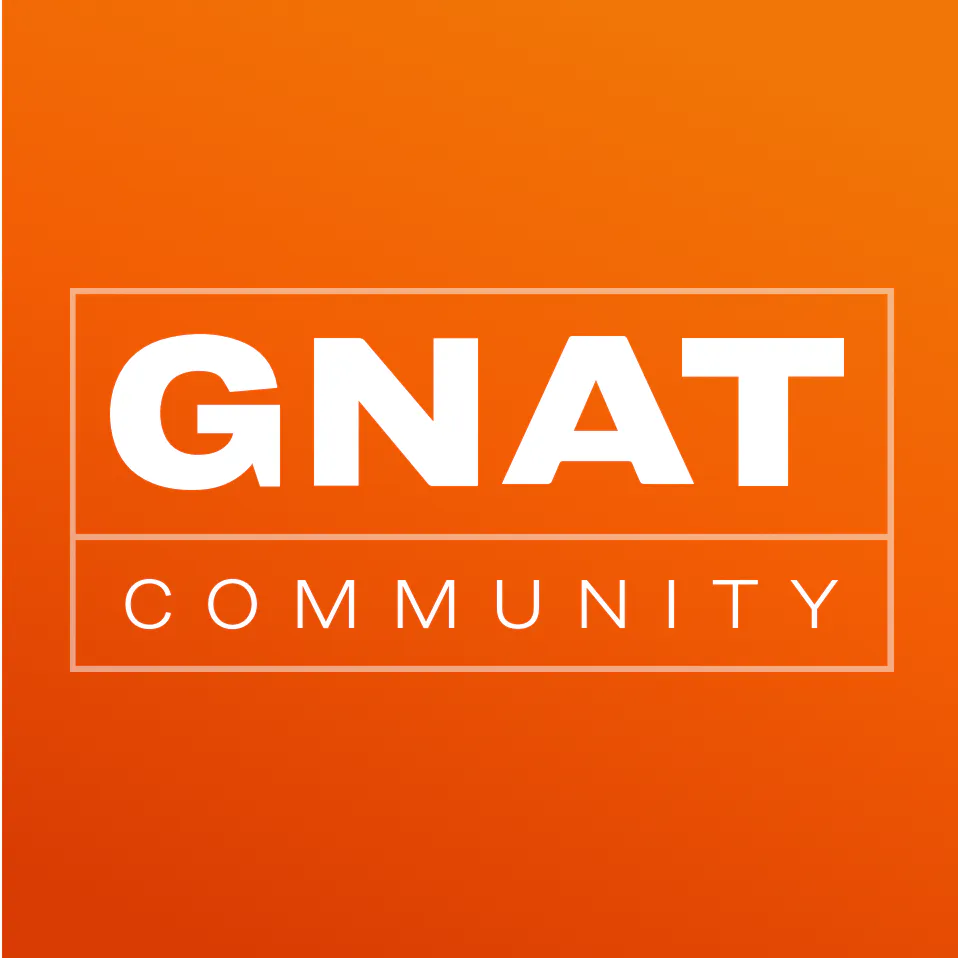This is a simple program to control the EEZYBOT MK2 robot arm using STM32F4 and Ada.

BeginnerWork in progress20 hours333Things used in this project

Hardware components

 STM32F407RGT6
×1SparkFun Stepper motor driver board A4988
×3Joystick, 10 kohm
×2

Software apps and online servicesHand tools and fabrication machines3D Printer (generic)

Custom parts and enclosures

EEZYBOT MK2 Stepper Version

The brilliant design for the robot arm can be found here. Credits to the designer.

Code

with XY_Driver;               pragma Unreferenced (XY_Driver);
with Last_Chance_Handler;  pragma Unreferenced (Last_Chance_Handler);
with System;
with Screen;

with HAL; use HAL;

with Rangesensor;

procedure Main is
pragma Priority (System.Priority'First);

Period : constant Time_Span := Milliseconds (400);
Next_Release : Time := Clock;

Y_Pos : Integer := 0;

begin
Screen.Init;
--  Rangesensor.Init;

loop
Screen.Put(X=> 50, Y=>24, Msg => Trim(Integer'Image(Y_Pos),Ada.Strings.Left));
Y_Pos := Y_Pos + 1;

if Y_Pos = 100 then
Screen.Clear_Screen;
Y_Pos := 0;
end if;

Next_Release := Next_Release + Period;
delay until Next_Release;

end loop;
end Main;

with STM32.Setup;

package body My_I2C is

function  Initialize return Boolean is
begin

STM32.Setup.Setup_I2C_Master (Port        => I2C_1,
SDA         => Screen_I2C_SDA,
SCL         => Screen_I2C_SCL,
SDA_AF      => GPIO_AF_I2C1_4,
SCL_AF      => GPIO_AF_I2C1_4,
Clock_Speed => 100_000);

return True;

end Initialize;

end My_I2C;

with STM32.GPIO;    use STM32.GPIO;
with STM32.Device;  use STM32.Device;

package My_I2C is
Screen_I2C_SDA : GPIO_Point renames PB7;
Screen_I2C_SCL : GPIO_Point renames PB6;

function Initialize return Boolean;

end My_I2C;

package body Rangesensor is

Status : Boolean := False;

procedure Init is
begin
null;
end Init;

begin

Initialize(My_Sensor);
Data_Init(This   => My_Sensor,
Status => Status);

if Status then

Static_Init(My_Sensor,GPIO_Function => Sensor_GPIO_Func,Status => Status);
end if;

if Status then
Perform_Ref_Calibration(This   => My_Sensor,
Status => Status);
end if;

if Status then
return UInt16(14);
else
return Uint16(22);

end if;

end Rangesensor;

with STM32.Device;  use STM32.Device;
with HAL.Time;
with HAL; use HAL;
with Ravenscar_Time;
with VL53L0X; use VL53L0X;

package Rangesensor is

Sensor_Id : UInt16 := 0;

HAL_Time : constant HAL.Time.Any_Delays := Ravenscar_Time.Delays;
My_Sensor : VL53L0X_Ranging_Sensor(I2C_1'Access,HAL_Time);
Sensor_GPIO_Func : VL53L0X_GPIO_Functionality := No_Interrupt;

procedure Init;

end Rangesensor;

with My_I2C;
with HAL.Bitmap; use HAL.Bitmap;
with Bitmapped_Drawing;
with Bitmap_Color_Conversion; use Bitmap_Color_Conversion;

package body Screen is

LCD_Natural_Width : constant Natural := 128;
LCD_Natural_Height : constant  Natural := 64;

Current_Font : constant BMP_Font := Default_Font;

Char_Width  : constant Natural := BMP_Fonts.Char_Width (Current_Font);
Char_Height : constant Natural := BMP_Fonts.Char_Height (Current_Font);

Max_Width   : constant Natural := LCD_Natural_Width - Char_Width;
--  The last place on the current "line" on the LCD where a char of the
--  current font size can be printed

Max_Height :constant  Natural := LCD_Natural_Height - Char_Height;
--  The last "line" on the LCD where a char of this current font size can be
--  printed

Current_Y : Natural := 0;
--  The current "line" that the text will appear upon. Note this wraps
--  around to the top of the screen.

Char_Count : Natural := 0;
--  The number of characters currently printed on the current line

procedure Init is
begin

if My_I2C.Initialize then
SSD1306.Initialize(My_Screen, External_VCC => False);
SSD1306.Initialize_Layer(This => My_Screen,
Layer =>1,
Mode=> M_1,
Height =>128,
Width => 64);
SSD1306.Turn_On(My_Screen);
SSD1306.Hidden_Buffer(My_Screen,1).Set_Source (Current_Background_Color);
SSD1306.Hidden_Buffer(My_Screen,1).Fill;
SSD1306.Update_Layer(My_Screen,1);

end if;

end Init;

procedure Clear_Screen is

begin
SSD1306.Hidden_Buffer(My_Screen,1).Set_Source (Current_Background_Color);
SSD1306.Hidden_Buffer(My_Screen,1).Fill;
Current_Y := 0;
Char_Count := 0;
SSD1306.Update_Layer(My_Screen,1);
end Clear_Screen;

procedure Draw_Char (X, Y : Natural; Msg : Character) is
begin
Bitmapped_Drawing.Draw_Char
(SSD1306.Hidden_Buffer(My_Screen,1).all,
Start      => (X, Y),
Char       => Msg,
Font       => Current_Font,
Foreground =>
Bitmap_Color_To_Word (M_1,
Current_Text_Color),
Background =>
Bitmap_Color_To_Word (M_1,
Current_Background_Color));
end Draw_Char;

procedure Internal_Put (Msg : Character) is
X : Natural;
begin
if Char_Count * Char_Width > Max_Width then
--  go to the next line down
Current_Y := Current_Y + Char_Height;
if Current_Y > Max_Height then
Current_Y := 0;
end if;
--  and start at beginning of the line
X := 0;
Char_Count := 0;
else
X := Char_Count * Char_Width;
end if;

Draw_Char (X, Current_Y, Msg);
Char_Count := Char_Count + 1;
end Internal_Put;

procedure Internal_Put (Msg : String) is

begin
for C of Msg loop
if C = ASCII.LF then
New_Line;
else
Internal_Put(C);
end if;
end loop;
end Internal_Put;

procedure Put (Msg : String) is
begin
Internal_Put (Msg);
SSD1306.Update_Layer(My_Screen,1);
end Put;

procedure Put (Msg : Character) is

begin
Internal_Put (Msg);
SSD1306.Update_Layer(My_Screen,1);
end Put;

procedure New_Line is
begin
Char_Count := 0; -- next char printed will be at the start of a new line
if Current_Y + Char_Height > Max_Height then
Current_Y := 0;
else
Current_Y := Current_Y + Char_Height;
end if;
end New_Line;

procedure Put_Line (Msg : String) is
begin
Put (Msg);
New_Line;
end Put_Line;

procedure Put (X, Y : Natural; Msg : Character) is
begin
Draw_Char (X, Y, Msg);
SSD1306.Update_Layer(My_Screen,1);
end Put;

---------
-- Put --
---------

procedure Put (X, Y : Natural; Msg : String) is
Count  : Natural := 0;
Next_X : Natural;
begin
for C of Msg loop
Next_X := X + Count * Char_Width;
Draw_Char (Next_X, Y, C);
Count := Count + 1;
end loop;

SSD1306.Update_Layer(My_Screen,1);
end Put;

end Screen;

with SSD1306; use SSD1306;
with STM32.Device; use STM32.Device;
with Ravenscar_Time;
with HAL.Time;
with BMP_Fonts;     use BMP_Fonts;
with HAL.Bitmap;
--with HAL.Framebuffer;

package Screen is

HAL_Time : constant HAL.Time.Any_Delays := Ravenscar_Time.Delays;
My_Screen : SSD1306_Screen((128 * 64) / 8, 128, 64,I2C_1'Access,PE3'Access, HAL_Time);

Black       : HAL.Bitmap.Bitmap_Color renames HAL.Bitmap.Black;
White       : HAL.Bitmap.Bitmap_Color renames HAL.Bitmap.White;

Default_Text_Color       : constant HAL.Bitmap.Bitmap_Color := White;
Default_Background_Color : constant HAL.Bitmap.Bitmap_Color := Black;
Default_Font             : constant BMP_Font := Font16x24;

Current_Text_Color       : HAL.Bitmap.Bitmap_Color := Default_Text_Color;
Current_Background_Color : HAL.Bitmap.Bitmap_Color := Default_Background_Color;

procedure Clear_Screen;

----------------------------------------------------------------------------

--  These routines maintain a logical line and column, such that text will
--  wrap around to the next "line" when necessary, as determined by the
--  current orientation of the screen.

procedure Put_Line (Msg : String);
--  Note: wraps around to the next line if necessary.
--  Always calls procedure New_Line automatically after printing the string.

procedure Put (Msg : String);
--  Note: wraps around to the next line if necessary.

procedure Put (Msg : Character);

procedure New_Line;
--  A subsequent call to Put or Put_Line will start printing characters at
--  the beginning of the next line, wrapping around to the top of the LCD
--  screen if necessary.

----------------------------------------------------------------------------

--  These routines are provided for convenience, as an alternative to
--  using both this package and an instance of Bitmnapped_Drawing directly,
--  when wanting both the wrap-around semantics and direct X/Y coordinate
--  control. You can combine calls to these routines with the ones above but
--  these do not update the logical line/column state, so more likely you
--  will use one set or the other. If you only need X/Y coordinate control,
--  consider directly using an instance of HAL.Bitmap.

procedure Put (X, Y : Natural; Msg : Character);
--  Prints the character at the specified location. Has no other effect
--  whatsoever, especially none on the state of the current logical line
--  or logical column.

procedure Put (X, Y : Natural; Msg : String);
--  Prints the string, starting at the specified location. Has no other
--  effect whatsoever, especially none on the state of the current logical
--  line or logical column. Does not wrap around.

procedure Init;

end Screen;

with STM32.Device;       use STM32.Device;
with STM32.GPIO;       use STM32.GPIO;
with HAL;          use HAL;

use STM32;

package body XY_Driver is

Period : constant Time_Span := Milliseconds (10);
Next_Release : Time := Clock;

X_drive : GPIO_Point renames PC4;
X_dir : GPIO_Point renames PC3;

Y_drive : GPIO_Point renames PB0;
Y_dir : GPIO_Point renames PA4;

Input_Channel_X : constant Analog_Input_Channel := 5;
Input_X         : constant GPIO_Point := PA5;

Input_Channel_Y : constant Analog_Input_Channel := 6;
Input_Y         : constant GPIO_Point := PA6;

All_Regular_X_Conversions : constant Regular_Channel_Conversions :=
(1 => (Channel => Input_Channel_X, Sample_Time => Sample_3_Cycles));

All_Regular_Y_Conversions : constant Regular_Channel_Conversions :=
(1 => (Channel => Input_Channel_Y, Sample_Time => Sample_3_Cycles));

Raw_X : UInt32 := 0;
Raw_Y : UInt32 := 0;

Successful : Boolean;

begin
Enable_Clock (X_drive & X_dir);
Configure_IO
(X_drive & X_dir,
(Mode_Out,
Resistors   => Floating,
Output_Type => Push_Pull,
Speed       => Speed_100MHz));

Enable_Clock (Y_drive );
Configure_IO
(Y_drive,
(Mode_Out,
Resistors   => Floating,
Output_Type => Push_Pull,
Speed       => Speed_100MHz));

Enable_Clock (Y_dir );
Configure_IO
(Y_dir,
(Mode_Out,
Resistors   => Floating,
Output_Type => Push_Pull,
Speed       => Speed_100MHz));

Enable_Clock (Input_X & Input_Y);
Configure_IO (Input_X & Input_Y, (Mode => Mode_Analog, Resistors => Floating));

Enable_Clock (X_Converter);
Enable_Clock (Y_Converter);

Configure_Common_Properties
(Mode           => Independent,
Prescalar      => PCLK2_Div_2,
DMA_Mode       => Disabled,
Sampling_Delay => Sampling_Delay_5_Cycles);

Configure_Unit
(X_Converter,
Alignment  => Right_Aligned);

Configure_Unit
(Y_Converter,
Alignment  => Right_Aligned);

Configure_Regular_Conversions
(X_Converter,
Continuous  => False,
Trigger     => Software_Triggered,
Enable_EOC  => True,
Conversions => All_Regular_X_Conversions);

Configure_Regular_Conversions
(Y_Converter,
Continuous  => False,
Trigger     => Software_Triggered,
Enable_EOC  => True,
Conversions => All_Regular_Y_Conversions);

Enable (X_Converter);
Enable (Y_Converter);

loop

Start_Conversion (X_Converter);
Poll_For_Status (X_Converter, Regular_Channel_Conversion_Complete, Successful);

if Successful then
Raw_X := UInt32(Conversion_Value (X_Converter));
if Raw_X < 1500 then
Set(X_dir);
Toggle (X_drive);
elsif Raw_X > 2500 then
Clear(X_dir);
Toggle (X_drive);
else
null;
end if;

end if;

Start_Conversion (Y_Converter);
Poll_For_Status (Y_Converter, Regular_Channel_Conversion_Complete, Successful);

if Successful then
Raw_Y := UInt32(Conversion_Value (Y_Converter));
if Raw_Y < 1500 then
Set(Y_dir);
Toggle (Y_drive);
elsif Raw_Y > 2500 then
Clear(Y_dir);
Toggle (Y_drive);
else
null;
end if;

end if;
Next_Release := Next_Release + Period;
delay until Next_Release;

end loop;
end XY_Controller;

end XY_Driver;

package XY_Driver is